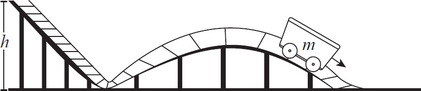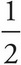# AP Physics 1 Question 315: Answer and Explanation

### Test Information

Question: 3153. An empty mine car of mass m starts at rest at the top of a hill of height h above the ground, then rolls down the hill and into a semicircular banked turn. Ignoring rolling friction so that the only forces acting on the mine car are the normal force from the track and gravity, what is the magnitude of centripetal force on the car as it rounds the banked curve?

• A. mgh
• B. 2mgh/r
• C. mgh/r
• D. mgh/(2r)

At the top of the ramp, the car will have potential energy PE = mgh. At the bottom, this same amount of energy has been converted into kinetic energy KE =mv2 = mgh. Multiplying both sides by 2 produces mv2 = 2mgh. Finally, divide both sides by r to get mv2/r = 2mgh/r. The first term is the general formula for centripetal force, so the second term must also be equivalent to that force in this situation.